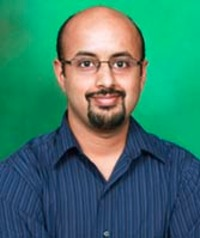Dr. Varadharaj R. SrinivasanAssociate Professor, Mathematical Sciences Email ravisri(AT)iisermohali.ac.inPhone +91 172 2293105 Fax +91 172 2240266 Personal Page Research AreaDifferential Algebra
 Research Focus My current research interests are in the areas of computational differential algebra, differential Galois theory and symbolic integration. In the 1970's and 1980's, several mathematicians (J. Ax, M. Bronstein, M. Rosenlicht, R. Risch, M. Singer) used techniques in differential algebra to study the structure of elementary field extensions and Liouvillian field extensions. The main goals are to study the problem of integration in finite terms and to understand certain number theoretic conjectures such as the Schanuel's conjecture.A major branch of differential algebra is the Galois theory of linear differential equations. Just as classical Galois theory addresses polynomial equations, differential Galois theory addresses linear homogeneous differential equations. The Galois groups that arise in this context happen to be linear algebraic groups. There is also a Galois correspondence in this context that works similarly to the Galois theory of polynomial equations. The nature of the solutions of a differential equation can be analyzed by studying the differential Galois group. For instance, all the solutions of a linear homogeneous differential equation are Liouvillian, that is, the solutions can be algebraically expressed by a combination of arbitrary antiderivatives, exponentials and algebraic elements if and only if the identity component of the differential Galois group is solvable. One can also apply differential Galois theory to determine whether or not an elementary function has an elementary integral. Selected Publications Iterated antiderivative extensions, Journal of Algebra, (8) 324 (2010) 2042-2051. (with William Keigher) Automorphisms of Hurwitz series, Homology, Homotopy and Applications (Accepted). Picard-Vessiot extensions for certain unipotent algebraic groups, submitted, arXiv: 1102. 3205v1 [math.CA]. Conference Proceedings (Peer Reviewed) (with William Keigher) Linear differential equations and Hurwitz series, Algebraic Methods for Dynamical Systems, Banach Center Publications Proceedings, (94) 2011.